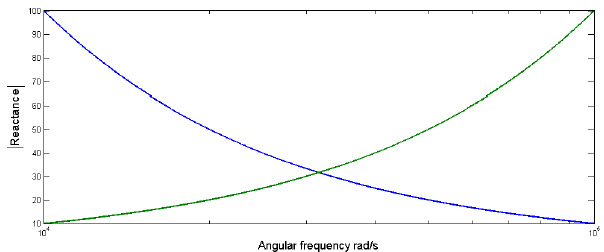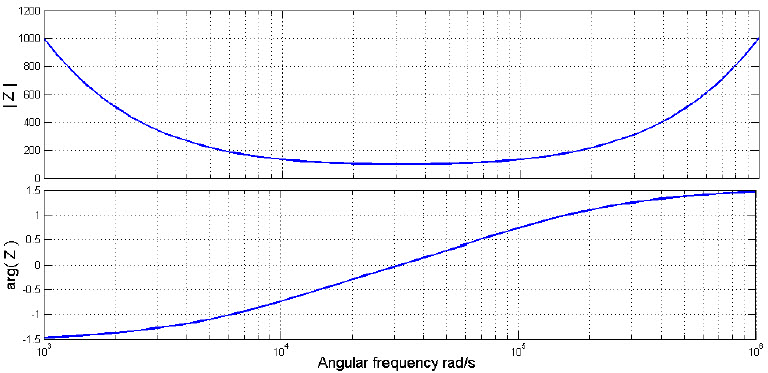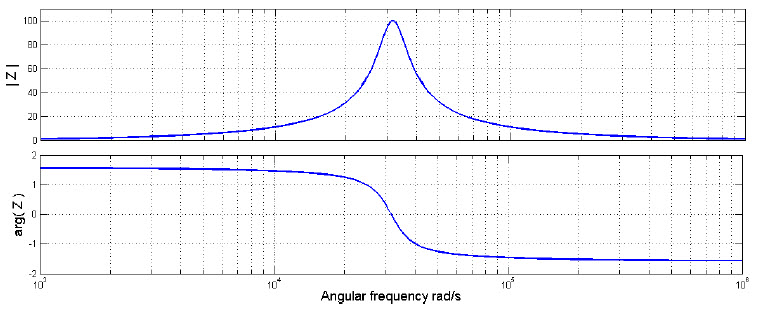# Guide on Resonant RLC Circuits Working and Applications

An RLC circuit is an electrical circuit it consists of a resistor, inductor, and capacitor they are represented by the letters R, L and C. The resonant RLC circuits are connected in series and parallel. The name RLC circuit is derived from the starting letter from the components of resistance, inductor, and capacitor. For the current purpose, the circuit forms a harmonic oscillator. Using the LC circuit it from resonates. If the resistor increases, it decomposes the oscillations it is known as damping. Some resistance is difficult to find in real-time, even after the resistor is not identified as the component it is solved by the LC circuit.

## Resonant RLC Circuits

While dealing with the resonant it is a complex component and it has a lot of discrepancies. The impedance z and its circuit are defined as

Z = R + JX

Where R is resistance, J is an imaginary unit and X is a reactance.

There is a pulse signed between R and JX. The imaginary unit is an outside resistance. The stored energy is the components of the capacitor and inductor. The capacitors are stored in the electrical field and inductors are stored in magnitude field.

ZC =1/ jωc

=-J/ωc

ZL = jωL

From the equation Z = R + JK we can define the reactances as

XC = -1/ωc

XL =ωL

The absolute value of the reactance of the inductor and capacitor charge with frequency as shown in the below figure.### Q Factor

The abbreviation of the Q is defined as a quality and it is also known as a Quality factor. The quality factor describes the under-damped resonator. If under-damped resonator increases the quality factor decreases. The electrical resonator circuit damping generates the loss of energy in resistive components. The mathematical expression of the Q factor is defined as the

Q(ω) = maximum power energy stored / power loss

The q factor is reliant on the frequency it is most frequently quote for the resonant frequency and the maximum energy stored in the capacitor and in the inductor can calculate the resonant frequency which is stored in the resonant circuit. The relevant equations are

Max energy stored =  LI2Lrms = C  V2Crms

ILrms are denoted as the RMS current through the inductor. It is equal to the total RMS current forming in the circuit in the series circuit and in the parallel circuit it is not equal. Similarly, in the VCrms is a voltage across the capacitor it is shown in the parallel circuit and it is equal to the rms supply voltage but in the series, the circuit is agreed by a potential divider. Thus the series circuit is simple to calculate the maximum energy stored through indicator and in the parallel circuits is considered through a capacitor.

Real power degenerates in the resistor

P = VRrms IRrms = I2Rrms R = V2Rrms/R

The easiest way to find the series RLC circuit

Q(S)ω0 = ω0  I2rms L/ I2rms R = ω0 L / R

The parallel circuit is to consider voltage

Q(P)ω0 = ω0 RCV2Crms / V2Crms = ω0 CR

### Series RLC Circuit

The RLC series circuit consists of resistance, inductor, and capacitor which are connected in series in the series RLC circuit. The below diagram shows the series RLC circuit. In this circuit capacitor and the inductor will combine each other and increase the frequency. If we can reconnect the Xcis a negative, so it is clear that XL + XC should be equal to zero for this specific frequency XL = -XCimpedance components of imaginary exactly cancel each other. At this frequency movement, the impedance of the circuit has low magnitude and phase angle of zero, it is called as the resonant frequency of the circuit.

XL + XC= 0

XL = – XC0L=1/ω0C = 1/LC

ω0 =√1/LC ω0

=  2Π f

### Arbitrary RLC Circuit

We can observe the resonance effects by considering the voltage across the resistive components to the input voltage for an example we can consider for the capacitor.

VC/V = 1/1-ω2LC + j ωRC

For the values of R, L, and C the ratio is plotted against angular frequency and the figure shows the properties of amplification. Resonant frequency

VC/V- 1/ j ω0RC

VC/V- j ω0L/R

We can see that as this is a positive circuit the total amount of power dissipated is constant### Parallel RLC Circuit

In the parallel RLC circuit the component’s resistance, inductor, and capacitor are connected in parallel. The resonant RLC circuit is a dual series circuit in the voltage and current exchange roles. Hence the circuit has a current gain rather than the impedance and the voltage gain is a maximum at the resonant frequency or minimized. The total impedance of the circuit is given as

= R ‖ ZL ‖ ZC

= R/ 1- JR (1/XC + 1/ XL)

= R / 1+ JR(ωc – 1/ωL)

When XC = – XLThe resonant peaks come once again and thus the resonant frequency has the same relationship.

ω0 =√1/LC

To calculate the current gain by looking the current in each of the arms, then the capacitor gain is given as

ic/i = jωRC/ 1+ jR(ωc – 1/ωL)The current gain of magnitude is shown in the figure and the resonant frequency is

ic/i= jRC

#### Applications of the Resonant RLC Circuits

The resonant RLC circuits have many applications like

• Oscillator circuit, radio receivers, and television sets are used for the tuning purpose.
• The series and RLC circuit mainly involves in signal processing and communication system
• The Series resonant LC circuit is used to provide voltage magnification
• Series and parallel LC circuit are used in induction heating

This article gives the information about RLC circuit, series and parallels RLC circuits, the Q factor, and applications of the resonant RLC circuits. I hope the given information in the article is helpful to give some good information and understanding the project. For furthermore, if you have any queries regarding this article or on the electrical and electronic projects you can comment in the below section. Here is a question for you, in parallel RLC circuit, which value may always be used as a vector reference?

Photo Credits: